• +91 9971497814
• info@interviewmaterial.com

# Chapter 9 Sequences and Series Ex-9.3 Interview Questions Answers

### Related Subjects

Question 1 : Find the 20th and nth terms of the G.P. 5/2, 5/4, 5/8, ………

Given G.P.is 5/2, 5/4, 5/8, ………

Here, a =First term = 5/2

r = Common ratio = (5/4)/(5/2) = ½

Thus, the 20th termand nth term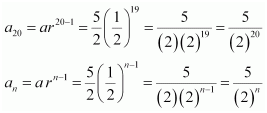Question 2 : Find the 12th term of a G.P.whose 8th term is 192 and the common ratio is 2.

Given,

The common ratio ofthe G.P., r = 2

And, let a bethe first term of the G.P.

Now,

a8 = ar 8–1 = ar7

ar7 = 192

a(2)7 = 192

a(2)7 = (2)6 (3)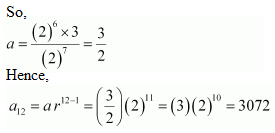Question 3 :

The 5th, 8th and 11th terms ofa G.P. are pq and s, respectively.Show that q2 = ps.

Let’s take a tobe the first term and r to be the common ratio of the G.P.

Then according to thequestion, we have

a5 = a r5–1 a r4 = p …(i)

aa r8–1 a r7 = q …(ii)

a11 = a r11–1 a r10 = …(iii)

Dividing equation (ii)by (i), we get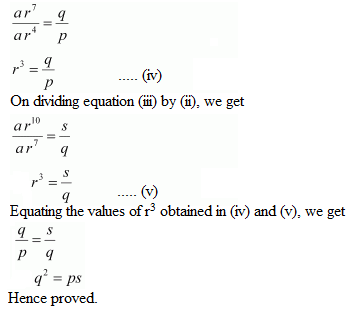Question 4 : The 4th term of a G.P. is square of its second term, and the first term is –3. Determine its 7th term.

Let’s consider a tobe the first term and r to be the common ratio of the G.P.

Given, a =–3

And we know that,

an = arn–1

So, aar3 =(–3) r3

a2 = a r1 = (–3) r

Then from thequestion, we have

(–3) r3 =[(–3) r]2

–3r3 =9 r2

r =–3

a7 = a r 7–1 a r6 =(–3) (–3)6 = – (3)7 = –2187

Therefore, the seventhterm of the G.P. is –2187.

Question 5 :

Which term of the following sequences:

(a) 2, 2√2, 4,… is 128 ? (b) √3, 3, 3√3,… is 729 ?

(c) 1/3, 1/9, 1/27, … is 1/19683 ?

(a) The givensequence, 2, 2√2, 4,…

We have,

a = 2 and r = 2√2/2 =√2

Taking the nth termof this sequence as 128, we have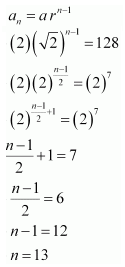Therefore, the 13th termof the given sequence is 128.

(ii) Given sequence,√3, 3, 3√3,…

We have,

a = √3 and r = 3/√3 =√3

Taking the nth termof this sequence to be 729, we have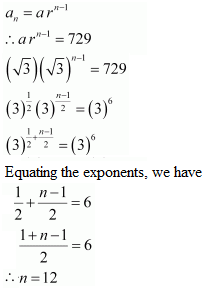Therefore, the 12th termof the given sequence is 729.

(iii) Given sequence,1/3, 1/9, 1/27, …

a = 1/3 and r =(1/9)/(1/3) = 1/3

Taking the nth termof this sequence to be 1/19683, we have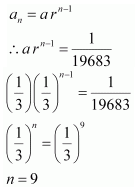Therefore, the 9th termof the given sequence is 1/19683.

Question 6 :

For what values of x, the numbers -2/7, x, -7/2 arein G.P?

The given numbers are-2/7, x, -7/2.

Common ratio =x/(-2/7) = -7x/2

Also, common ratio= (-7/2)/x = -7/2x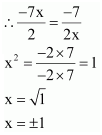Therefore, for x =± 1, the given numbers will be in G.P.

Question 7 :

Find the sum to 20 terms in the geometric progression 0.15, 0.015, 0.0015…

Given G.P., 0.15,0.015, 0.00015, …

Here, a =0.15 and r = 0.015/0.15 = 0.1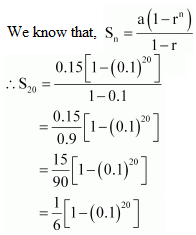Question 8 :

Find the sum to n terms in the geometric progression √7,√21, 3√7, ….

The given G.P is √7,√21, 3√7, ….

Here,

a = √7 and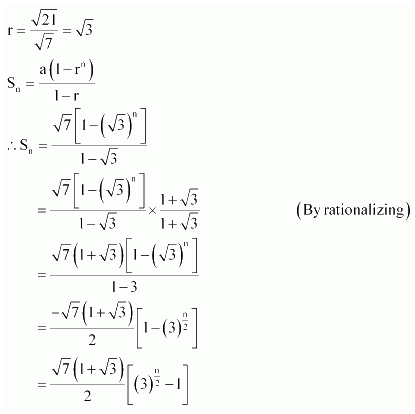Question 9 : Find the sum to n terms inthe geometric progression 1, -a, a2, -a3 …. (if a ≠-1)

The given G.P.is 1, -a, a2, -a3 ….

Here, the first term= a1 = 1

And the common ratio= r = – a

We know that,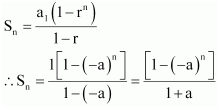Question 10 : Find the sum to n terms inthe geometric progression x3, x5, x7, … (if x≠ ±1 )

Given G.P. is x3,x5, x7, …

Here, we have a = x3 and r = x5/x3 =x2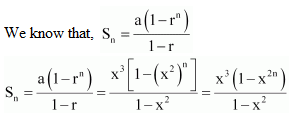Todays Deals### Chapter 9 Sequences and Series Ex-9.3 Contributorskrishan

Name:
Email:

# Latest News# 9000 interview questions in different categories